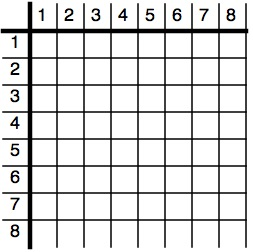#### You may also like### Prompt Cards

These two group activities use mathematical reasoning - one is numerical, one geometric.### Consecutive Numbers

An investigation involving adding and subtracting sets of consecutive numbers. Lots to find out, lots to explore.### Exploring Wild & Wonderful Number Patterns

EWWNP means Exploring Wild and Wonderful Number Patterns Created by Yourself! Investigate what happens if we create number patterns using some simple rules.

# Sometimes We Lose Things

##### Age 7 to 11Challenge Level

Some people say "Well it's just about being tidy", but sometimes things just seem to disappear!

We all use ten very useful things that come in very handy for all sorts of things. We use numerals for counting, and if you're used to NRICH Challenges, you use them for exploring.

Well now, what would happen if we lost one of these numerals or digits as we call them sometimes? It could be that we lost all the nines.

Or maybe back in history early man decided to count in nines instead of tens.

We would have to count:-and then we would have to say "one lot of nine and no units"

so we'd have

$10$

BUT this $10$ would NOT be read as the word TEN instead we would say NINE.

[You might notice that when, before we lost it, we had the number $9$ we would say TEN when we saw $10$, but we did not have a single symbol for TEN.]

So now we've lost the digit for NINE and so we have to use $10$ for NINE.

So counting further we have to go:-

write say
$1$ one
$2$ two
$3$ three
$4$ four
$5$ five
$6$ six
$7$ seven
$8$ eight
$10$ nine
$11$ nine one
$12$ nine two
$13$ nine three
$14$ nine four
$15$ nine five
$16$ nine six
$17$ nine seven
$18$ nine eight
$20$ two nines
$21$ two nines one
$22$ two nines two

Much further on if we were counting all the small squares on a chess board we would have:-

$71$ seven nines, one

If we were counting the small squares on a snakes and ladder board you would have:-

$121$ one (nine lots of nine), two nines, one

Well this is such a different thing to look at we should have a look a bit further. BUT FIRST have a go at writing the numbers out in this way, and then writing down how we could say it [like I have above from $1$ to $22$] and carry on until you've reached the number of years that have gone by in this century.

So going further we will have a look at the multiplications table. You might like to have a go on your own.

So start off with this:-You could find help here.

Now here's a table of numbers for you to investigate patterns. Have a look at the relationships between various numbers.

Remember to talk about the numbers in a new way, so that the last number is "seven nines and one". [NOT "seven tens and one" or more usually as "seventy one".]

There's not a lot more I want to say. Except that what ever you find out do send those findings to us because we'd like to be able to share them with others across the world!

The simplest of things will interest us.

I always ask you to carry on further by asking questions like:-

"I wonder what would happen if I ..."

You might, for example ask, "I wonder what would happen if the $8$ got lost as well as the $9$?" So we would go:- $1, 2, 3, 4, 5, 6, 7, 10, 11, 12$ to count our fingers and thumbs.

Another example might be "What if when we lost the $9$ we had a look at some big addition calculations?" like :-

        24 + 36 + 78 + 65 + 2



or maybe you'd prefer it as


24

36

78

65

2

___

___



You should get the answer $227$ [that is two (nine nines), two nines and seven]

I invite you to invent some good "I wonder what would happen if I ...?" questions and follow those questions further.

Good luck.